# 3-D Schrodinger Equation

In three dimensions, the time-independent Schrodinger equation takes the form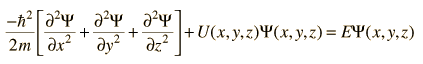for cartesian coordinates. This can be written in a more compact form by making use of the Laplacian operator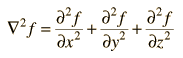The Schrodinger equation can then be written: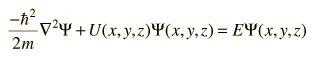For systems with a spherically symmetric potential, like the hydrogen atom, it is advantageous to use spherical coordinates.

Index

Schrodinger equation concepts
HyperPhysics***** Quantum Physics Go Back

# Schrodinger Equation, Spherical Coordinates

If the potential of the physical system to be examined is spherically symmetric, then the Schrodinger equation in spherical polar coordinates can be used to advantage. For a three-dimensional problem, the Laplacian in spherical polar coordinates is used to express the Schrodinger equation in the condensed form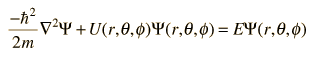Expanded, it takes the form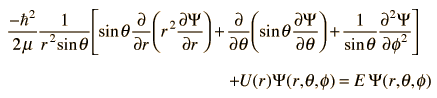This is the form best suited for the study of the hydrogen atom.
Index

Schrodinger equation concepts
HyperPhysics***** Quantum Physics Go Back